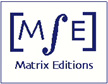Serious mathematics, written with the reader in mind.

Matrix Editions

Current Books

Future Books

Errata

Math in literature

Other Books

;

 Review from Zentralblatt MATH Teichmüller Theory and Applications to Geometry, Topology, and Dynamics   Volume I: Teichmüller Theory"The subject of Teichmüller theory is the study of moduli of Riemann surfaces. This subject has interconnections and applications in several areas in mathematics which inslude, besides complex analysis and hyperbolic geometry,  the theory of representation of discrete groups theory, algebraic geometry,  low-dimensional manifolds,  symplectic geometry, dynamical systems, number theory, topological quantum field theory, string theory (and there are others). Teichmüller theory was founded by Teichmüller in the 1930s,  with techniques that come from geometric complex analysis, and it was developed  on these grounds, during the three decades that followed Teichmüller's death, by Ahlfors, Bers and others. In the 1970s, Thurston introduced in that theory beautiful new techniques of hyperbolic geometry. His approach highlighted Teichmüller theory as a central object in the filed of low-dimensional topology. The book under review is the first volume in a series of two. It gives a comprehensive treatment  of the foundations of Teichmüller theory with its two aspects, complex analysis and hyperbolic geometry. The second part in the series will cover four  applications of Teichmüller theory to low-dimensional topology which were discovered by Thurston,  namely,  the classification of homeomorphisms of surfaces, the topological classification of rational maps,  the hyperbolization theorem for 3-manifolds that fiber over the circle and the hyperbolization of Haken 3-manifolds. Volume 1 of Hubbard's book covers all the important classical aspects of Teichmüller's theory. It includes beautiful treatments of classical theorems such as Poincaré's  uniformization theorem, a development of the theory of quasiconformal maps and of the solution to Beltrami's partial differential equation, a study of the space of quadratic differentials, of Schwarzian derivatives and of boundary values of extremal maps, and a beautiful treatment of  models of the hyperbolic plane, hyperbolic trigonometry, fundamental domains,  the Fenchel-Nielsen parametrization of hyperbolic surfaces, and the Weil-Petersson geometry of Teichmüller space. The book also includes treatments of more specialized topics that one can hardly find (and, for some of them, cannot find at all)  in other textbooks on Teichmüller theory. We mention here  Mumford's compactness theorem,  the Douady-Earle barycentric extension theory, the theory of holomorphic motions, Slodkowski's theorem, Royden's results on the auto- morphisms of Teichmüller spaces, the author's  work on sections of the universal Teichmüller curve and Wolpert's formula for the Weil-Petersson symplectic form. This is an invaluable  book. It treats  a wonderful subject, and it is written by a great mathematician. It is now an essential reference for every student and every researcher in the field." — Athanase Papadopoulos (Zentralblatt MATH 1102.30001)